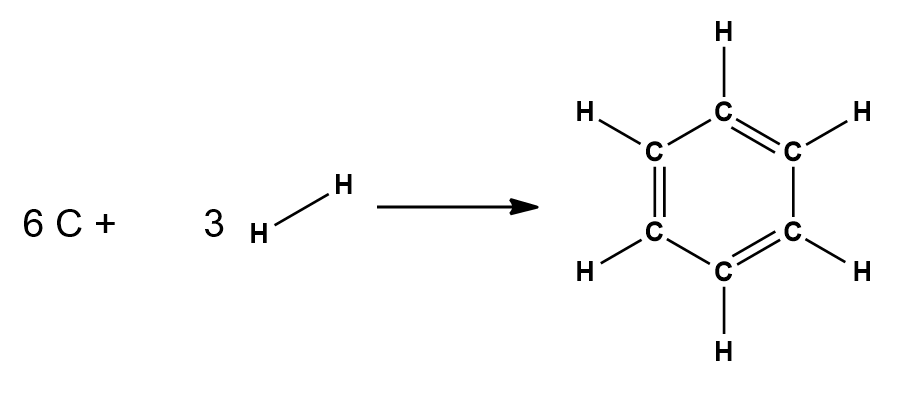# Problem: Use average bond energies together with the standard enthalpy of formation of C(g) (718.4 kJ/mol ) to estimate the standard enthalpy of formation of gaseous benzene, C6H6(g). (Remember that average bond energies apply to the gas phase only.)

###### FREE Expert Solution

For this problem, we are being asked to estimate the standard enthalpy of formation of gaseous benzene, C6H6(g)

Step 1. Write the balanced equation

6 C (s) + 3 H(g) → C6H6 (g)Step 2. Calculate the entalphy for formation

Recall that the change of enthalpy for a chemical reaction (ΔH) can be calculated using bond energies:

To determine the enthalpy of reaction, we need to first see what bonds are present in the reactants and the products.

95% (479 ratings)###### Problem Details

Use average bond energies together with the standard enthalpy of formation of C(g) (718.4 kJ/mol ) to estimate the standard enthalpy of formation of gaseous benzene, C6H6(g). (Remember that average bond energies apply to the gas phase only.)

Frequently Asked Questions

What scientific concept do you need to know in order to solve this problem?

Our tutors have indicated that to solve this problem you will need to apply the Bond Energy concept. You can view video lessons to learn Bond Energy. Or if you need more Bond Energy practice, you can also practice Bond Energy practice problems.

What professor is this problem relevant for?

Based on our data, we think this problem is relevant for Professor Roychowdhury's class at RUTGERS.